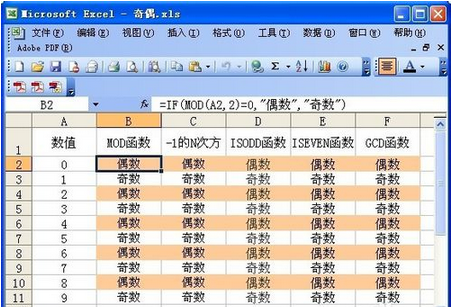# Excel中if函数，奇/偶数的设定是什么?

• A+

IF函数+MOD函数，公式：=IF(MOD(A2,2)=0,"偶数","奇数")；1．IF函数的语法结构

### 问题内容

Excel中if函数，奇/偶数的设定是什么? IF函数+MOD函数，公式：=IF(MOD(A2,2)=0,"偶数","奇数")；

### 网友回答

IF函数+MOD函数，公式：=IF(MOD(A2,2)=0,"偶数","奇数")；1．IF函数的语法结构

IF函数的语法结构：IF(条件,结果1,结果2)。

2．IF函数的功能

3．条件表达式

4．执行过程

5．IF函数嵌套的执行过程

IF(E2>=85,"优",IF(E2>=75,"良"，IF(E2>=60,"及格"，"不及格")))